# Unit ix graph

19. Sep 2015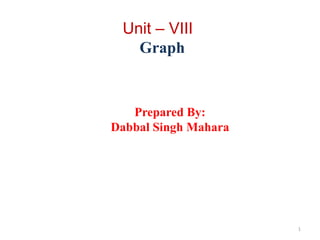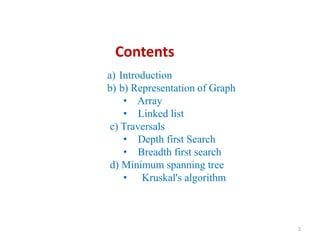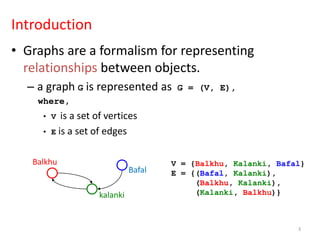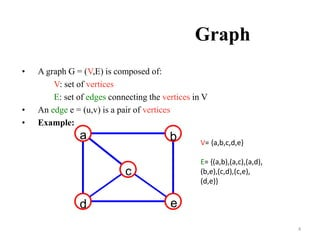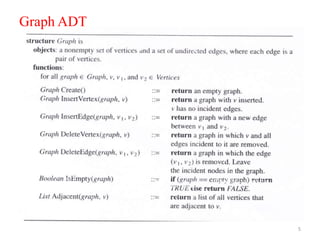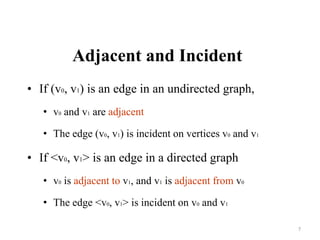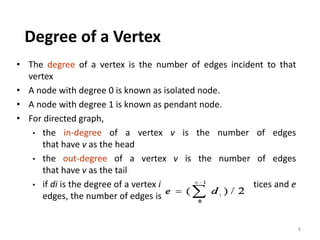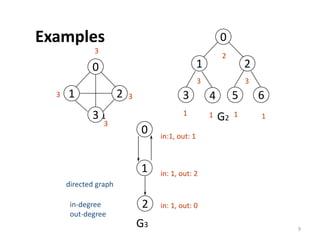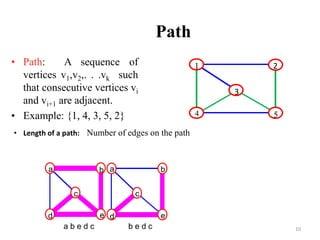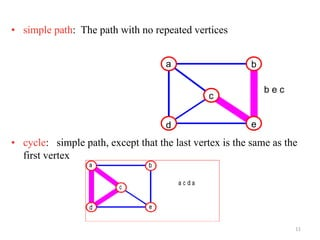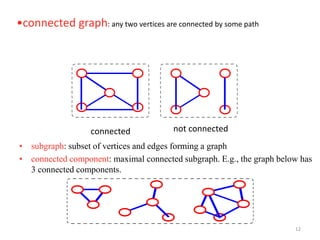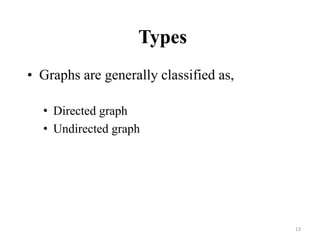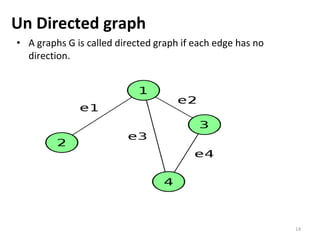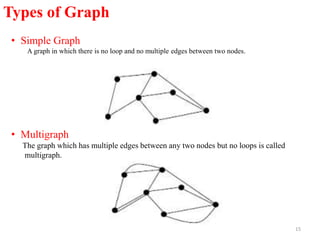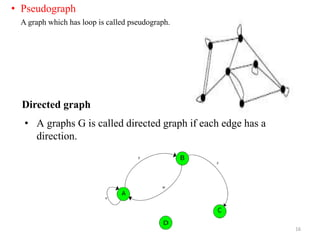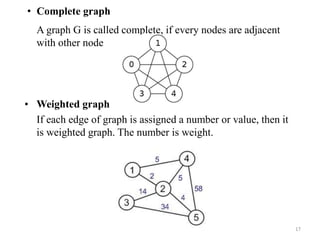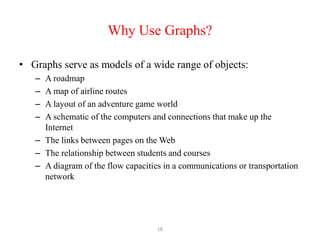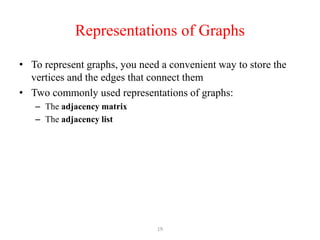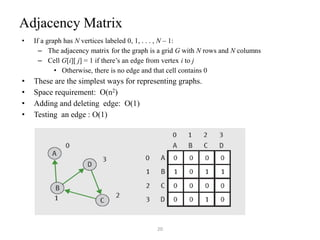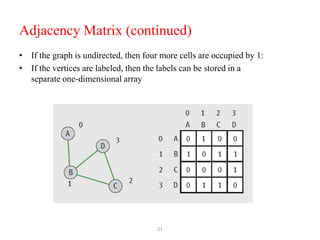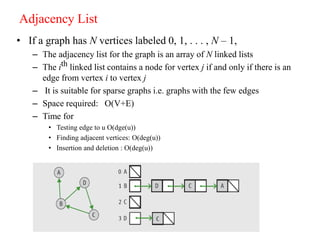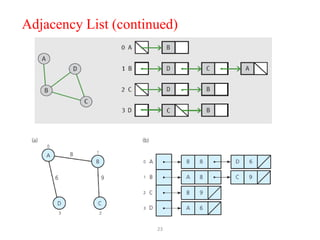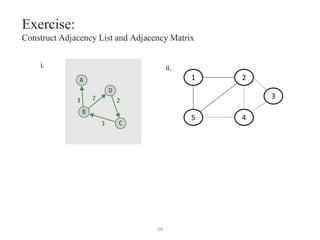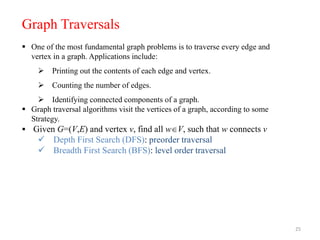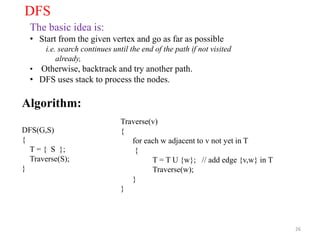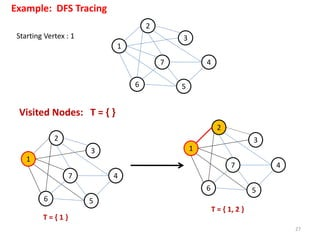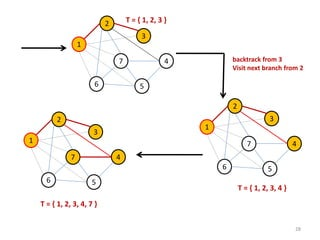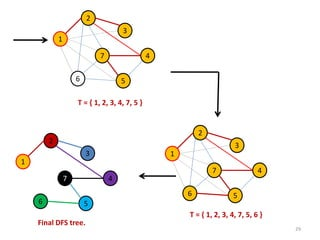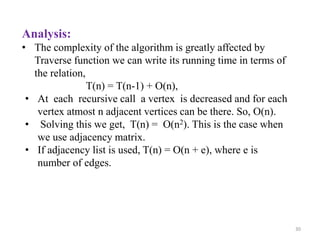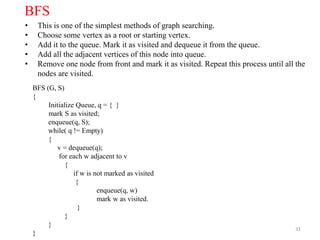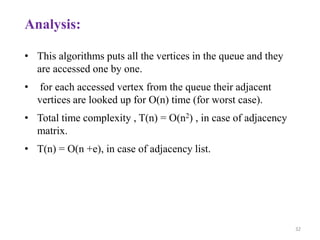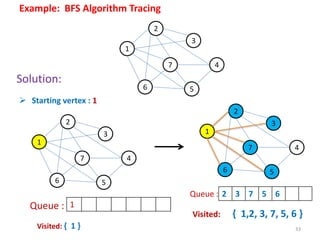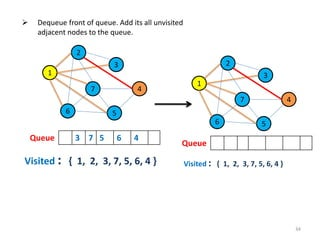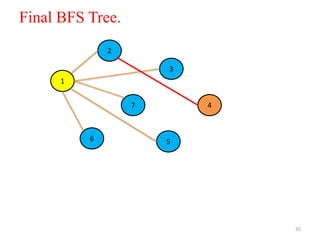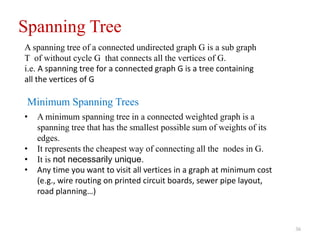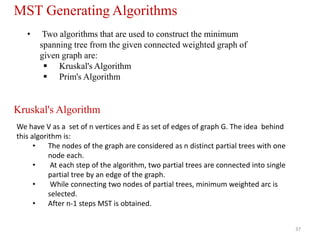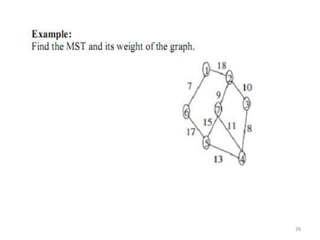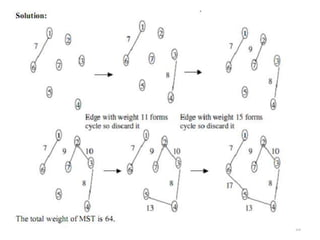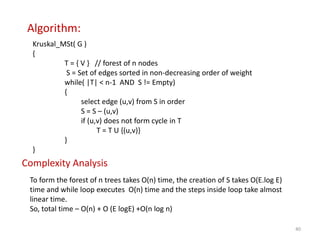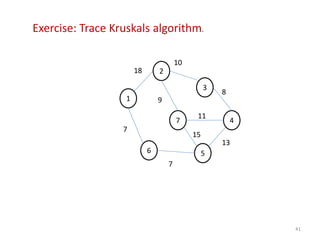1 von 42

### Unit ix graph

• 1. Unit – VIII Graph Prepared By: Dabbal Singh Mahara 1
• 2. 2 Contents a) Introduction b) b) Representation of Graph • Array • Linked list c) Traversals • Depth first Search • Breadth first search d) Minimum spanning tree • Kruskal's algorithm
• 3. Introduction • Graphs are a formalism for representing relationships between objects. – a graph G is represented as G = (V, E), where, • V is a set of vertices • E is a set of edges Balkhu kalanki Bafal V = {Balkhu, Kalanki, Bafal} E = {(Bafal, Kalanki), (Balkhu, Kalanki), (Kalanki, Balkhu)} 3
• 4. Graph • A graph G = (V,E) is composed of: V: set of vertices E: set of edges connecting the vertices in V • An edge e = (u,v) is a pair of vertices • Example: a b c d e V= {a,b,c,d,e} E= {(a,b),(a,c),(a,d), (b,e),(c,d),(c,e), (d,e)} 4
• 6. Graph Terminology: • Node Each element of a graph is called node of a graph • Edge Line joining two nodes is called an edge. It is denoted by e=[u,v] where u and v are adjacent vertices. 6  Loop An edge of the form (u, u) is said to be a loop. Here in figure [v2,v2]  Multiedge If x was y’s friend several times over, we can model this relationship using multiedges. In figure e1 and e3. Loop e1 V1 V2e2V2 node edge e3
• 7. Adjacent and Incident • If (v0, v1) is an edge in an undirected graph, • v0 and v1 are adjacent • The edge (v0, v1) is incident on vertices v0 and v1 • If <v0, v1> is an edge in a directed graph • v0 is adjacent to v1, and v1 is adjacent from v0 • The edge <v0, v1> is incident on v0 and v1 7
• 8. • The degree of a vertex is the number of edges incident to that vertex • A node with degree 0 is known as isolated node. • A node with degree 1 is known as pendant node. • For directed graph, • the in-degree of a vertex v is the number of edges that have v as the head • the out-degree of a vertex v is the number of edges that have v as the tail • if di is the degree of a vertex i in a graph G with n vertices and e edges, the number of edges is Degree of a Vertex 8
• 9. 0 1 2 3 4 5 6 G1 G2 3 2 3 3 1 1 1 1 directed graph in-degree out-degree 0 1 2 G3 in:1, out: 1 in: 1, out: 2 in: 1, out: 0 0 1 2 3 33 3 Examples 9
• 10. 10 Path • Path: A sequence of vertices v1,v2,. . .vk such that consecutive vertices vi and vi+1 are adjacent. • Example: {1, 4, 3, 5, 2} 1 4 5 3 2 a b c d e a b c d e a b e d c b e d c • Length of a path: Number of edges on the path
• 11. • simple path: The path with no repeated vertices • cycle: simple path, except that the last vertex is the same as the first vertex a b c d e b e c 11
• 12. • subgraph: subset of vertices and edges forming a graph • connected component: maximal connected subgraph. E.g., the graph below has 3 connected components. connected not connected •connected graph: any two vertices are connected by some path 12
• 13. Types • Graphs are generally classified as, • Directed graph • Undirected graph 13
• 14. Un Directed graph • A graphs G is called directed graph if each edge has no direction. 14
• 15. 15 • Simple Graph A graph in which there is no loop and no multiple edges between two nodes. • Multigraph The graph which has multiple edges between any two nodes but no loops is called multigraph. Types of Graph
• 16. Directed graph • A graphs G is called directed graph if each edge has a direction. 16 • Pseudograph A graph which has loop is called pseudograph.
• 17. • Complete graph A graph G is called complete, if every nodes are adjacent with other node • Weighted graph If each edge of graph is assigned a number or value, then it is weighted graph. The number is weight. 17
• 18. 18 Why Use Graphs? • Graphs serve as models of a wide range of objects: – A roadmap – A map of airline routes – A layout of an adventure game world – A schematic of the computers and connections that make up the Internet – The links between pages on the Web – The relationship between students and courses – A diagram of the flow capacities in a communications or transportation network
• 19. 19 Representations of Graphs • To represent graphs, you need a convenient way to store the vertices and the edges that connect them • Two commonly used representations of graphs: – The adjacency matrix – The adjacency list
• 20. 20 Adjacency Matrix • If a graph has N vertices labeled 0, 1, . . . , N – 1: – The adjacency matrix for the graph is a grid G with N rows and N columns – Cell G[i][ j] = 1 if there’s an edge from vertex i to j • Otherwise, there is no edge and that cell contains 0 • These are the simplest ways for representing graphs. • Space requirement: O(n2) • Adding and deleting edge: O(1) • Testing an edge : O(1)
• 21. 21 Adjacency Matrix (continued) • If the graph is undirected, then four more cells are occupied by 1: • If the vertices are labeled, then the labels can be stored in a separate one-dimensional array
• 22. 22 Adjacency List • If a graph has N vertices labeled 0, 1, . . . , N – 1, – The adjacency list for the graph is an array of N linked lists – The ith linked list contains a node for vertex j if and only if there is an edge from vertex i to vertex j – It is suitable for sparse graphs i.e. graphs with the few edges – Space required: O(V+E) – Time for • Testing edge to u O(dge(u)) • Finding adjacent vertices: O(deg(u)) • Insertion and deletion : O(deg(u))
• 24. 24 Exercise: Construct Adjacency List and Adjacency Matrix i. ii. 1 5 4 3 2
• 25. 25  One of the most fundamental graph problems is to traverse every edge and vertex in a graph. Applications include:  Printing out the contents of each edge and vertex.  Counting the number of edges.  Identifying connected components of a graph.  Graph traversal algorithms visit the vertices of a graph, according to some Strategy.  Given G=(V,E) and vertex v, find all wV, such that w connects v  Depth First Search (DFS): preorder traversal  Breadth First Search (BFS): level order traversal Graph Traversals
• 26. 26 DFS The basic idea is: • Start from the given vertex and go as far as possible i.e. search continues until the end of the path if not visited already, • Otherwise, backtrack and try another path. • DFS uses stack to process the nodes. Algorithm: DFS(G,S) { T = { S }; Traverse(S); } Traverse(v) { for each w adjacent to v not yet in T { T = T U {w}; // add edge {v,w} in T Traverse(w); } }
• 27. 27 2 1 6 7 5 3 4 Example: DFS Tracing Starting Vertex : 1 2 1 6 7 5 3 4 Visited Nodes: T = { } 2 1 6 7 5 3 4 T = { 1 } T = { 1, 2 }
• 28. 28 2 1 6 7 5 3 4 T = { 1, 2, 3 } backtrack from 3 Visit next branch from 2 2 1 6 7 5 3 4 T = { 1, 2, 3, 4 } 2 1 6 7 5 3 4 T = { 1, 2, 3, 4, 7 }
• 29. 29 T = { 1, 2, 3, 4, 7, 5 } 2 1 6 7 5 3 4 2 1 6 7 5 3 4 T = { 1, 2, 3, 4, 7, 5, 6 } 2 1 6 7 5 3 4 Final DFS tree.
• 30. 30 Analysis: • The complexity of the algorithm is greatly affected by Traverse function we can write its running time in terms of the relation, T(n) = T(n-1) + O(n), • At each recursive call a vertex is decreased and for each vertex atmost n adjacent vertices can be there. So, O(n). • Solving this we get, T(n) = O(n2). This is the case when we use adjacency matrix. • If adjacency list is used, T(n) = O(n + e), where e is number of edges.
• 31. 31 BFS • This is one of the simplest methods of graph searching. • Choose some vertex as a root or starting vertex. • Add it to the queue. Mark it as visited and dequeue it from the queue. • Add all the adjacent vertices of this node into queue. • Remove one node from front and mark it as visited. Repeat this process until all the nodes are visited. BFS (G, S) { Initialize Queue, q = { } mark S as visited; enqueue(q, S); while( q != Empty) { v = dequeue(q); for each w adjacent to v { if w is not marked as visited { enqueue(q, w) mark w as visited. } } } }
• 32. 32 Analysis: • This algorithms puts all the vertices in the queue and they are accessed one by one. • for each accessed vertex from the queue their adjacent vertices are looked up for O(n) time (for worst case). • Total time complexity , T(n) = O(n2) , in case of adjacency matrix. • T(n) = O(n +e), in case of adjacency list.
• 33. 33 2 1 6 7 5 3 4 Example: BFS Algorithm Tracing Solution: 2 1 6 7 5 3 4  Starting vertex : 1 Visited: { 1 } 2 1 6 7 5 3 4 Visited: { 1,2, 3, 7, 5, 6 } 1 2 3 7 5 6Queue : Queue :
• 34. 34 2 1 6 7 5 3 4  Dequeue front of queue. Add its all unvisited adjacent nodes to the queue. Queue 3 7 5 6 4 Visited : { 1, 2, 3, 7, 5, 6, 4 } 2 1 6 7 5 3 4 Queue Visited : { 1, 2, 3, 7, 5, 6, 4 }
• 36. 36 Spanning Tree A spanning tree of a connected undirected graph G is a sub graph T of without cycle G that connects all the vertices of G. i.e. A spanning tree for a connected graph G is a tree containing all the vertices of G • A minimum spanning tree in a connected weighted graph is a spanning tree that has the smallest possible sum of weights of its edges. • It represents the cheapest way of connecting all the nodes in G. • It is not necessarily unique. • Any time you want to visit all vertices in a graph at minimum cost (e.g., wire routing on printed circuit boards, sewer pipe layout, road planning…) Minimum Spanning Trees
• 37. 37 • Two algorithms that are used to construct the minimum spanning tree from the given connected weighted graph of given graph are:  Kruskal's Algorithm  Prim's Algorithm MST Generating Algorithms Kruskal's Algorithm We have V as a set of n vertices and E as set of edges of graph G. The idea behind this algorithm is: • The nodes of the graph are considered as n distinct partial trees with one node each. • At each step of the algorithm, two partial trees are connected into single partial tree by an edge of the graph. • While connecting two nodes of partial trees, minimum weighted arc is selected. • After n-1 steps MST is obtained.
• 38. 38
• 39. 39
• 40. 40 Algorithm: Kruskal_MSt( G ) { T = { V } // forest of n nodes S = Set of edges sorted in non-decreasing order of weight while( |T| < n-1 AND S != Empty) { select edge (u,v) from S in order S = S – (u,v) if (u,v) does not form cycle in T T = T U {(u,v)} } } Complexity Analysis To form the forest of n trees takes O(n) time, the creation of S takes O(E.log E) time and while loop executes O(n) time and the steps inside loop take almost linear time. So, total time – O(n) + O (E logE) +O(n log n)
• 42. 42